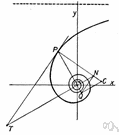# hyperbolic geometry

Also found in: Thesaurus, Encyclopedia, Wikipedia.
ThesaurusAntonymsRelated WordsSynonymsLegend:
 Noun 1hyperbolic geometry - (mathematics) a non-Euclidean geometry in which the parallel axiom is replaced by the assumption that through any point in a plane there are two or more lines that do not intersect a given line in the plane; "Karl Gauss pioneered hyperbolic geometry"math, mathematics, maths - a science (or group of related sciences) dealing with the logic of quantity and shape and arrangementnon-Euclidean geometry - (mathematics) geometry based on axioms different from Euclid's; "non-Euclidean geometries discard or replace one or more of the Euclidean axioms"
Mentioned in ?
References in periodicals archive ?
From a July-August 2014 institute of the Mathematical Society of Japan in Tokyo, 13 papers consider hyperbolic geometry and geometric group theory from such perspectives as the recognition problem for virtually special cube complexes, a classification of radial or totally geodesic ends of real projective orbifolds: a survey of results, Abelian splitting of right-angled Artin groups, the hyperbolic analogues of some classical theorems in spherical geometry, and a note on acylindrical hyperbolicity of mapping class groups.
Goldman, Complex hyperbolic geometry, Oxford Mathematical Monographs, The Clarendon Press, Oxford University Press, New York, 1999.
Hyperbolic geometry does indeed rest on an axiom not in Euclid's system, but it has been shown by Poincare and others that if Euclidian geometry is true and consistent, so is hyperbolic geometry.
Mirzakhani's research focuses on hyperbolic geometry.
De Las Penas is interested in hyperbolic geometry and tilings, and has written about weaving maths, symmetry in handwoven mats, and weaving symmetry in the Kankanaey of northern Philippines.
A characterization of inscribable 3-poly topes was found by Hodgson, Rivin and Smith using hyperbolic geometry [HRS92, Riv96].
Six survey and expository papers and 11 research papers explore hyperbolic geometry, symplectic geometry, geometric topology, and other areas of geometry and topology.
Hyperbolic Geometry appeared in the first half of the 19th century as an attempt to understand Euclid's axiomatic basis of Geometry.
CAIDA researcher Dmitri Krioukov, along with Marian Boguna and Fragkiskos Papadopoulos, have described how they discovered a latent hyperbolic, or negatively curved, space hidden beneath the Internet's topology, leading them to devise a method to create an Internet map using hyperbolic geometry.
These colloquia held every 3-4 years are dedicated to the contributions of the late mathematicians Lars Ahlfors and Lipman Bers to the fields of geometric function theory, hyperbolic geometry, partial differential equations, and Teichmuller theory.
Today, the non-Euclidean geometry of Gauss, Lobachevsky and Bolyai is called hyperbolic geometry, and the term non- Euclidean refers to any geometry that is not Euclidean.
Ungar, Analytic Hyperbolic Geometry and Albert Einstein's Special Theory of Relativity, Hackensack, NJ: World Scientific Publishing Co.

Site: Follow: Share:
Open / Close